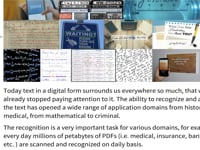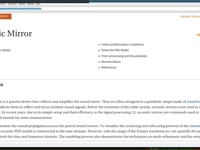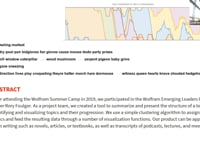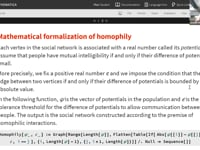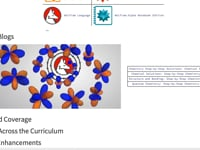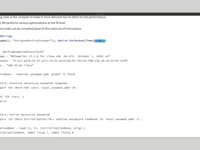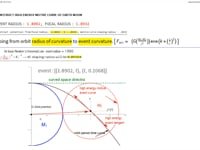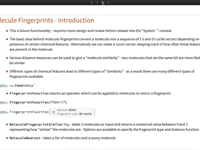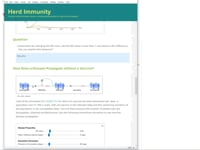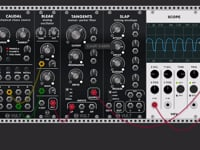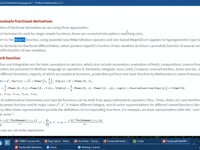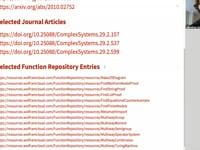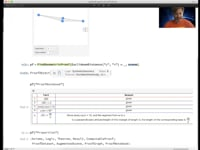Learn how »

# Modeling Multi-physics with PDEs

In this talk, Oliver Ruebenkoenig describes how to build multi physics models in the Wolfram Language to simulate multiple interacting physical phenomena. It introduces a new partial differential equation (PDE) modeling language that makes it easy to set up both PDEs and boundary conditions. Basic building blocks and advanced PDE models are shown, as well as an introduction to augmenting your equations with the PDE model terms. The mathematical models are based on systems of possibly nonlinear PDEs that can be solved numerically using NDSolve and NDEigensystem. This talk will provide you with the capabilities to build real-world PDE models involving, but not limited to, acoustics, heat/mass transfer, solid mechanics and fluid dynamics.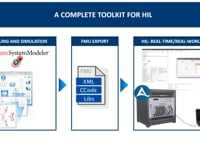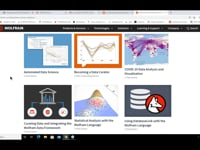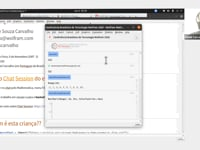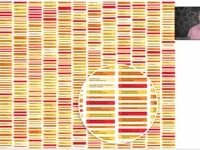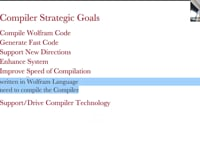The Wolfram Compiler61:14